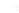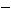To add decimals, write the numbers in a column, and make sure that the decimal points are aligned. To keep the decimal point in your sum, place a decimal point directly below the decimal points in the addends.

Example

Solve: 0.07 + 2.8 + 0.5

Solution

Align the decimal point in the sum with decimal point in the addends, add a zero if necessary. Then add the numbers from right to left.

+ 0.07+ 0.07+ 0.07+ 01.07

+ 2.80+ 2.80+ 2.80+ 2 .80

+ 0.50+ 0.50+ 0.50+ 0 .50

+ 0.50+ 0.57+ 0.37+ 3 .37

Thus, 0.07 + 2.8 + 0.5 = 3.37

Estimation

When using a calculator to add decimals, estimate the sum by rounding each number to the same place value or to the nearest whole number. You can then check the calculator's result against your estimate.

Subtraction with Decimals

To subtract decimals, begin by writing the numbers in a column, with the decimal points aligned. If necessary, add zeroes as place holders to make sure there are values in each column. Subtract from right to left. Write the decimal point in the difference directly below the decimal point in the subtrahend. You can check the answer by adding.

Example

Solve: 46.28.75

Use the decimal points to line up the digits, add zeroes as needed

46.208.75

First subtract the hundredths

10

46.208.75

37.45

then subtract the tenths

11

46.208.75

37.45

finally subtract the ones and tens

15

46.208.75

37.45

3

46.208.75

37.45

Then 46.28.75 = 37.45

Estimation

When using a calculator to find the difference between decimals, first estimate the difference between the numbers by rounding to the same place value (or nearest whole number). Then check your calculator's result with your estimate to make sure it is reasonable.